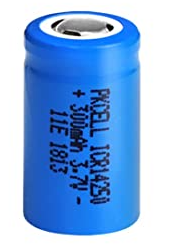﻿ Joule's Law Calculator ﻿# Joule's Law CalculatorNote: The current or resistance value cannot be zero

 Enter the Current, I A (amperes) mA (milliamperes) µA (microamperes) Enter the Resistance, R: Ω (ohms) mΩ (milliohms) Enter the Time, t: s (seconds) mS (Milliseconds) µS (Microseconds)

Energy Dissipated as Heat:

This Joule's law calculator calculates the energy dissipated as heat when an electric current passes through a resistance per unit period of time.

1 Joule is equal to the energy dissipated as heat when an electric current of one ampere passes through a resistance of one ohm for one second.

Joule's law applies to batteries, because every battery has some internal resistance due to a battery not being a perfect conductor and its inherent internal composition and makeup.

Current is the flow of electrons.

When the electrons pass through the internal resistance of the battery, there is friction and this produces heat.

This heat produces power loss in the circuit.

This power loss dissipated as heat is calculated according to the formula, Energy= I2Rt, where I is the current passing through the battery, R is the internal resistance of the battery, and t is time.

Usually the internal resistance of a lithium ion battery is just a few milliohms. The best batteries will have the lowest internal resistance, as the lower the internal resistance, the less heat is generated, thus, the less power loss there is through heat dissipation due to the internal resistance.

Lithium ion batteries may have an internal resistance ranging from 5-30 milliohms.

Thus, for example, if there is 15mA passing through a battery with 5 milliohms in 1 second, the battery will dissipate 0.000001125 joules per second.

Related Resources

﻿